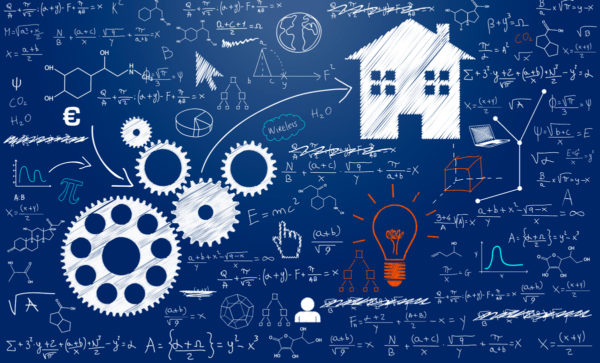# News# CCC3 Crosscutting Concept 3 – Scale, Proportion, and Quantity

In considering phenomena, it is critical to recognize what is relevant at different measures of size, time, and energy and to recognize how changes in scale, proportion, or quantity affect a system’s structure or performance.

Scale, Proportion and Quantity are important in both science and engineering. These are fundamental assessments of dimension that form the foundation of observations about nature. Before an analysis of function or process can be made (the how or why), it is necessary to identify the what. These concepts are the starting point for scientific understanding, whether it is of a total system or its individual components. Any student who has ever played the game “twenty questions” understands this inherently, asking questions such as, “Is it bigger than a bread box?” in order to first determine the object’s size.

An understanding of scale involves not only understanding systems and processes vary in size, time span, and energy, but also different mechanisms operate at different scales. In engineering, “no structure could be conceived, much less constructed, without the engineer’s precise sense of scale... At a basic level, in order to identify something as bigger or smaller than something else—and how much bigger or smaller—a student must appreciate the units used to measure it and develop a feel for quantity.” (p. 90)

“The ideas of ratio and proportionality as used in science can extend and challenge students’ mathematical understanding of these concepts. To appreciate the relative magnitude of some properties or processes, it may be necessary to grasp the relationships among different types of quantities—for example, speed as the ratio of distance traveled to time taken, density as a ratio of mass to volume. This use of ratio is quite different than a ratio of numbers describing fractions of a pie. Recognition of such relationships among different quantities is a key step in forming mathematical models that interpret scientific data.” (p. 90)

The crosscutting concept of Scale, Proportion, and Quantity figures prominently in the practices of “Using Mathematics and Computational Thinking” and in “Analyzing and Interpreting Data.” This concept addresses taking measurements of structures and phenomena, and these fundamental observations are usually obtained, analyzed, and interpreted quantitatively. This crosscutting concept also figures prominently in the practice of “Developing and Using Models.” Scale and proportion are often best understood using models. For example, the relative scales of objects in the solar system or of the components of an atom are difficult to comprehend mathematically (because the numbers involved are either so large or so small), but visual or conceptual models make them much more understandable (e.g., if the solar system were the size of a penny, the Milky Way galaxy would be the size of Texas).

source: http://www.nextgenscience.org/